## Example Questions

### Example Question #1 : How To Simplify A Fraction

Simplify the following fraction: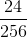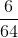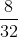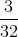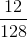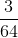Explanation:

Find the largest number that divides into bothand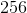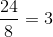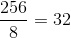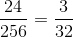### Example Question #2 : How To Simplify A Fraction

Simplify the following fraction until the numerator and denominator share no factors.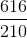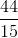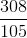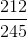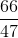Explanation:

To simplify a fraction you need to find all the factors that the numerator and denominator have in common. You can see that both share 2 so when you divide both by 2 you getthis is close to the answer but it asks for no common factors, it is hard to see but both of these share 7 as a common factor. When you divide both by 7 you get### Example Question #3 : How To Simplify A Fraction

Which of the following is the least common denominator for the expression below?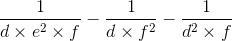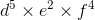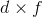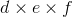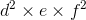Explanation:

Finding the least common denominator in rational expressions follows the same procedue as finding the least common denominator in fractions.

The least common denominator for this rational exresspion will use all terms with the highest exponents of each.

The first fraction has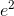as the highest term, the second fraction has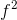as the highest term, and the third fraction has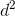as the highest term. Now we combine these and get the least common denominator to be: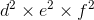### Example Question #4 : How To Simplify A Fraction

Simplify the following fraction: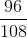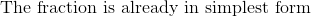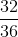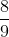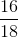Explanation:

To simplify a fraction, find the gcf (gcd) of the numerator and denominator and divide both by the gcf (gcd). the gcf ofand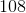isso: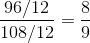### Example Question #5 : How To Simplify A Fraction

Simplify the following fraction: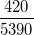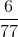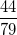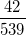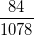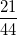Explanation:

The first and easiest simplification for this fraction is to divide numerator and denominator by.  This gives you:Next, notice that the two fractions do not both contain factors of.  This is because the denominator's digits, when added up come to, which is not divisible by.  This means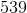is not divisible by.  Now,is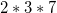.  There is no sharedbetween these numbers.  However, if you try, you will see that they are both divisible by, which gives you:This is simplest form.

### Example Question #6 : How To Simplify A Fraction

Simplify the following fraction: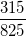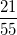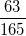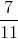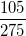Explanation:

First, begin by noticing that both numerator and denominator contain a. Dividing this out gives you:Now,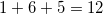and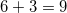.  Since each of these are true, we know that both numerator and denominator contain a.  Dividing this out, you get:This is the simplest possible form of the fraction.

### Example Question #7 : How To Simplify A Fraction

Maria owns an art studio and spent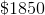in supplies.  She sells her paintings for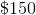each.  How many paintings does Maria need to sell until she makes a profit?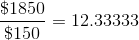Therefore, to make a profit, she needs to sell more than this amount.  Since she can't sell a portion of a painting, the answer has to be the next nearest whole number ().